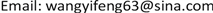1. 引言

2. 光子质量、能量和动量三者之间的关系

E = M c 2 (2)

E = h c λ = h v (3)

1   J = 1   kg ⋅ ( m / s ) 2 (4)

M = h λ c (5)

E c = M c (7)

p = M c (8)

M c = p = h λ (9)

3. 光子的波长和频率是没有物理意义的辅助变量

4. 从普朗克常数的角度看不确定性原理和物质波概念之间的矛盾

( x + Δ x ) ⋅ ( p + Δ p ) = x ⋅ p + x ⋅ Δ p + Δ x ⋅ p + Δ x ⋅ Δ p (15)

x ⋅ p > x ⋅ Δ p > Δ x ⋅ p > Δ x ⋅ Δ p

{ x ⋅ p ≥ h x ⋅ Δ p ≥ h Δ x ⋅ p ≥ h Δ x ⋅ Δ p ≥ h Δ x ≠ 0 , Δ p ≠ 0 (16)

Δ x ⋅ Δ p ≥ h (17)

p + Δ p = ( m + Δ m ) ⋅ ( u + Δ u ) = m ⋅ u + m ⋅ Δ u + Δ m ⋅ u + Δ m ⋅ Δ u (18)

( x + Δ x ) ⋅ ( p + Δ p ) = ( x + Δ x ) ⋅ ( m + Δ m ) ⋅ ( u + Δ u ) = x ⋅ m ⋅ u + x ⋅ m ⋅ Δ u + x ⋅ Δ m ⋅ u + x ⋅ Δ m ⋅ Δ u     + Δ x ⋅ m ⋅ u + Δ x ⋅ m ⋅ Δ u + Δ x ⋅ Δ m ⋅ u + Δ x ⋅ Δ m ⋅ Δ u (19)

{ x ⋅ m ⋅ u ≥ h x ⋅ m ⋅ Δ u ≥ h x ⋅ Δ m ⋅ u ≥ h x ⋅ Δ m ⋅ Δ u ≥ h Δ x ⋅ m ⋅ u ≥ h Δ x ⋅ m ⋅ Δ u ≥ h Δ x ⋅ Δ m ⋅ u ≥ h Δ x ⋅ Δ m ⋅ Δ u ≥ h Δ x ≠ 0 , Δ m ≠ 0 , Δ u ≠ 0 (20)

Δ x ⋅ Δ m ⋅ Δ u ≥ h (21)

{ x ⋅ m ⋅ u ≥ h x ⋅ m ⋅ Δ u ≥ h Δ x ⋅ m ⋅ u ≥ h Δ x ⋅ m ⋅ Δ u ≥ h Δ x ≠ 0 , Δ u ≠ 0 (22)

Δ x ⋅ m ⋅ Δ u ≥ h (23)

Δm = 0实际上引入了质量不变或者质量守恒的概念。但是不能因为质量守恒就认为质量没有不确定性，因为毕竟质量具体有多少也是测量出来的。所以广义的不确定性原理应该采用式(21)。

( x + Δ x ) ⋅ ( p + Δ p ) = x ⋅ p = h (24)

x = h p (25)

p = h x (26)

λ = h p (27)

p = h λ (28)

λ p = h (29)

p = M c (30)

M = h λ c (31)

M = E c 2 (32)

E = h c λ = h v (33)

5. 结束语

“物质波以及波动现象在量子论里有较大的作用  (第46页)”，另一方面，不确定性原理“是量子力学的组成部分，同时也是它的天然而直接的结论  (第57页)”。如果剔掉物质波概念，再加上不确定性原理没有多少物理意义，量子力学理论的基础或将被动摇。